# Python線性數據結構

python線性數據結構 [TOC] 1 線性數據結構 本章要介紹的線性結構：list、tuple、string、bytes、bytearray。 線性表：是一種抽象的數學概念，是一組元素的序列的抽象，由有窮個元素組成(0個或任意個)。 線性表又可分為 順序表和鏈接表。 順序表：一組元素在記憶體中有序 ...

python線性數據結構

``````<center>碼好python的每一篇文章.</center>
``````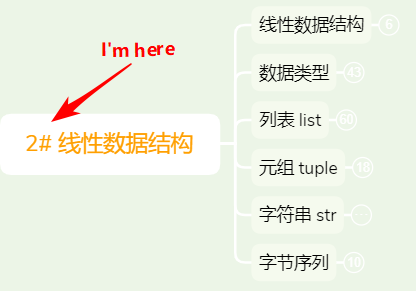# 1 線性數據結構

• 線性表：是一種抽象的數學概念，是一組元素的序列的抽象，由有窮個元素組成(0個或任意個)。

線性表又可分為 順序表和鏈接表。

• 順序表：一組元素在記憶體中有序的存儲。列表list就是典型的順序表。

• 鏈接表：一組元素在記憶體中分散存儲鏈接起來，彼此知道連接的是誰。

對於這兩種表，數組中的元素進行查找、增加、刪除、修改，看看有什麼影響：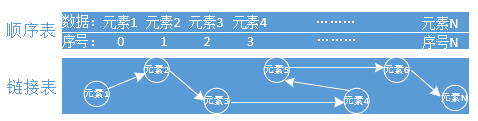• 查找元素

對於順序表，是有序的在記憶體中存儲數據，可快速通過索引編號獲取元素，效率高。。

對於鏈接表是分散存儲的，只能通過一個個去迭代獲取元素，效率差。

• 增加元素

對於順序表，如果是在末尾增加元素，對於整個數據表來說沒什麼影響，但是在開頭或是中間插入元素，後面的所有元素都要重新排序，影響很大(想想數百萬或更大數據量)。

對於鏈接表，不管在哪裡加入元素，不會影響其他元素，影響小。

• 刪除元素
對於順序表，刪除元素和增加元素有著一樣的問題。
對於鏈接表，不管在哪裡刪除元素，不會影響其他元素，影響小。

• 修改元素
對於順序表，可快速通過索引獲取元素然後進行修改，效率高。

對於鏈接表，只能通過迭代獲取元素然後進行修改，效率低。

# 2.內建常用的數據類型

## 2.1 數值型

• int 整數類型

說明：整數包括負整數、0、正整數（... -2,-1,0,1,2, ...）。

``````x1 = 1
x2 = 0
x3 = -1
print(type(x1), x1)
print(type(x2), x2)
print(type(x3), x3)

# 輸出結果如下：
<class 'int'> 1
<class 'int'> 0
<class 'int'> -1
``````

int( )方法：可以將數字或字元串轉為整數，預設base=10,表示10進位，無參數傳入則返回0。

``````x1 = int()
x2 = int('1')
x3 = int('0b10',base=2)  #base=2，表二進位，與傳入參數類型一致。
x4 = int(3.14)
print(type(x1), x1)
print(type(x2), x2)
print(type(x3), x3)
print(type(x4), x4)

# 輸出結果如下：
<class 'int'> 0
<class 'int'> 1
<class 'int'> 2
<class 'int'> 3
``````
• float 浮點類型
說明：由整數和小數部分組成，傳入的參數可以為`int``str``bytes``bytearray`

``````x1 = float(1)
x2 = float('2')
x3 = float(b'3')
print(type(x1), x1)
print(type(x2), x2)
print(type(x3), x3)

# 輸出結果如下：
<class 'float'> 1.0
<class 'float'> 2.0
<class 'float'> 3.0
``````
• complex (複數類型)

說明：由實數和虛數部分組成，都是浮點數。

傳入參數可以為`int``str`，如果傳入兩參，前面一個為實數部分，後一個參數為虛數部分。

``````x1 = complex(1)
x2 = complex(2,2)
x3 = complex('3')
print(type(x1), x1)
print(type(x2), x2)
print(type(x3), x3)

# 輸出結果如下：
<class 'complex'> (1+0j)
<class 'complex'> (2+2j)
<class 'complex'> (3+0j)
``````
• bool (布爾類型)

說明：為int的子類，返回的是True和False，對應的是1和0。

``````x1 = bool(0)
x2 = bool(1)
x3 = bool()
x4 = 2 > 1
print(type(x1), x1)
print(type(x2), x2)
print(type(x3), x3)
print(type(x4), x4)

# 輸出結果如下：
<class 'bool'> False
<class 'bool'> True
<class 'bool'> False
<class 'bool'> True
``````

## 2.2 序列(sequence)

### 2.2.1 list 列表

• 初始化

``````lst = []  # 空列表方式1
#或者
lst = list()  # 空列表方式2
print(type(lst),lst)

# 輸入結果如下：
<class 'list'> []
``````
• 索引

說明： 使用正索引(從左至右)、負索引(從右至左)訪問元素，時間複雜度為`O(1)`，效率極高的使用方式。

按照給定區間獲取到數據，叫做切片。

正索引：

從左至右，從0開始索引，區間為[0，長度-1]，左包右不包。

``````lst = ['a','b','c','d']
print(lst)  # 獲取第一個元素
print(lst[1:2])  # 獲取第二個元素,左包右不包，切片
print(lst[2:])  # 獲取第三個元素到最後一個元素，切片
print(lst[:])  # 獲取所有元素，切片

# 輸出結果如下：
a
['c']
['c', 'd']
['a', 'b', 'c', 'd']
``````

負索引：

從右至左，從-1開始索引，區間為[-長度，-1]

``````lst = ['a','b','c','d']
print(lst[-1])
print(lst[-2:])

# 輸出結果如下：
d
['c', 'd']
``````
• 查詢

index( )方法：`L.index(value, [start, [stop]]) -> integer`

返回的是索引id，要迭代列表，時間複雜度為O(n)。

``````lst = ['a','b','c','d']
print(lst.index('a',0,4))  # 獲取區間[0,4]的元素'a'的索引id

# 輸出結果如下：
0
``````

備註：如果查詢不到元素，則拋出`ValueError`

count( ) 方法：L.count(value) -> integer

返回的是元素出現的次數，要迭代列表，時間複雜度為O(n)。

``````lst = ['a','b','a','b']
print(lst.count('a'))

# 輸出結果如下：
2
``````

len( ) 方法：返回的是列表元素的個數，時間複雜度為O(1)。

``````lst = ['a','b','c','d']
print(len(lst))

# 輸出結果如下：
4
``````

備註：所謂的O(n) 是指隨著數據的規模越來越大，效率下降，而O(1)則相反，不會隨著數據規模大而影響效率。

• 修改

列表是有序可變，所以能夠對列表中的元素進行修改。

``````lst = ['a','b','c','d']
lst = 'A'
print(lst)

# 輸出結果如下：
['A', 'b', 'c', 'd']
``````
• 增加

append( ) 方法：`L.append(object) -> None`

尾部追加元素，就地修改，返回None。

``````lst = ['a','b','c','d']
lst.append('e')
print(lst)

# 輸出結果如下：
['a', 'b', 'c', 'd', 'e']
``````

insert( )方法：`L.insert(index, object) -> None` ,

在指定索引位置插入元素對象，返回None。

``````lst = ['a','b','c','d']
lst.insert(0,'A')  # 在索引0位置插入'A',原有的元素全部往後移，增加了複雜度
print(lst)

# 輸出結果如下：
['A', 'a', 'b', 'c', 'd']
``````

extend( )方法： `L.extend(iterable) -> None`

可以增加多個元素，將可迭代對象的元素追加進去，返回None。

``````lst = ['a','b','c','d']
lst.extend([1,2,3])
print(lst)

# 輸出結果如下：
['a', 'b', 'c', 'd', 1, 2, 3]
``````

還可以將列表通過 `+``*` ，拼接成新的列表。

``````lst1 = ['a','b','c','d']
lst2 = ['e','f','g']
print(lst1 + lst2)
print(lst1 * 2)  # 將列表裡面的元素各複製2份

# 輸出結果如下：
['a', 'b', 'c', 'd', 'e', 'f', 'g']
['a', 'b', 'c', 'd', 'a', 'b', 'c', 'd']
``````

這裡還有一個特別要註意情況如下：

``````lst1 = [] * 3  # 結果：[, , ]
print(lst1)
lst1 = 10  # 結果：[, , ]，是這樣嘛？？
print(lst1)

# 輸出結果如下：
[, , ]
[, , ]  # 為什麼結果會是這個？請往下看列表複製章節，找答案！
``````
• 刪除

remove()方法：`L.remove(value) -> None`

從左至右遍歷查找，找到就刪除該元素，返回None，找不到則拋出`ValueError`

``````lst = ['a','b','c','d']
lst.remove('d')
print(lst)

# 輸出結果如下：
['a', 'b', 'c']  # 元素'd'已經被刪除
``````

pop() 方法：`L.pop([index]) -> item`

預設刪除尾部元素，可指定索引刪除元素，索引越界拋出`IndexError`

``````lst = ['a','b','c','d']
lst.pop()
print(lst)

# 輸出結果如下：
['a', 'b', 'c']
``````

clear() 方法：`L.clear() -> None`

清空列表所有元素，慎用。

``````lst = ['a','b','c','d']
lst.clear()
print(lst)

# 輸出結果如下：
[]  # 空列表了
``````
• 反轉

reverse( ) 方法：`L.reverse()`

將列表中的元素反轉，返回None。

``````lst = ['a','b','c','d']
lst.reverse()
print(lst)

# 輸出結果如下：
['d', 'c', 'b', 'a']
``````
• 排序

sort() 方法：`L.sort(key=None, reverse=False) -> None`

對列表元素進行排序，預設為升序，reverse=True為降序。

``````lst = ['a','b','c','d']
lst.sort(reverse=True)
print(lst)

# 輸出結果如下：
['d', 'c', 'b', 'a']
``````
• in成員操作

判斷成員是否在列表裡面，有則返回True、無則返回False。

``````lst = ['a','b','c','d']
print('a' in lst)
print('e' in lst)

# 輸出結果如下：
True
False
``````
• 列表複製

說明： 列表複製指的是列表元素的複製，可分為淺copy和深copy兩種。列表元素對象如列表、元組、字典、類、實例這些歸為引用類型(指向記憶體地址)，而數字、字元串先歸為簡單類型，好讓大家理解。

示例一：這是屬於拷貝嘛？

``````lst1 = [1,[2,3],4]
lst2 = lst1
print(id(lst1),id(lst2),lst1 == lst2, lst2)  # id() 查看記憶體地址

# 輸出結果如下：
1593751168840 1593751168840 True [1, [2, 3], 4]
``````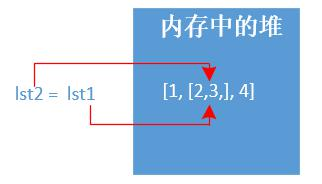顯然不是屬於任何copy，說白了都是指向同一個記憶體地址。

示例二：淺拷貝copy

說明： 淺拷貝對於`引用類型`對象是不會copy的，地址指向仍是一樣。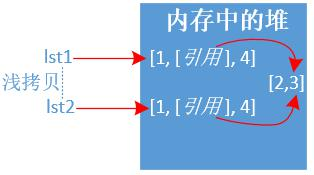``````lst1 = [1,[2,3],4]
lst2 = lst1.copy()
print(id(lst1),id(lst2),lst1 == lst2, lst2)
print('=' * 30)
lst1 = 200  # 修改列表的引用類型，所有列表都會改變
print(lst1, lst2)

# 輸出結果如下：
1922175854408 1922175854344 True [1, [2, 3], 4]
==============================
[1, [200, 3], 4] [1, [200, 3], 4]
``````

示例三：深拷貝deepcopy

說明： 深拷貝對於`引用類型`對象也會copy成另外一份，地址指向不一樣。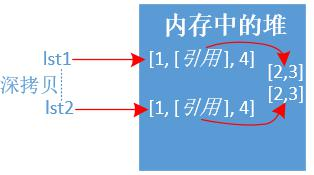``````import copy

lst1 = [1,[2,3],4]
lst2 = copy.deepcopy(lst1)
print(id(lst1),id(lst2),lst1 == lst2, lst2)
print('=' * 30)
lst1 = 200  # 修改列表的引用類型，不會影響其他列表
print(lst1, lst2)

# 輸出結果如下：
2378580158344 2378580158280 True [1, [2, 3], 4]
==============================
[1, [200, 3], 4] [1, [2, 3], 4]
``````

### 2.2.2 tuple 元組

• 初始化

``````t1 = ()  # 空元素方式1，一旦創建將不可改變
t2 = tuple()  # 空元素方式2，一旦創建將不可改變
t3 = ('a',)  # 元組只有一個元素，一定要加逗號','
t4 = (['a','b','c'])  # 空列表方式2
``````

備註： 元組如果只有一個元素對象，一定要在後面加逗號`,` 否則變為其他數據類型。

• 索引
同列表一樣，不再過多舉例。

``````t = ('a','b','c','d')
print(t)
print(t[-1])
# 輸出結果如下：
a
d
``````
• 查詢

同列表一樣，不再過多舉例。

``````t = ('a','b','c','d')
print(t.index('a'))
print(t.count('a'))
print(len(t))

# 輸出結果如下：
0
1
4
``````
• 增刪改

元組是`不可變`類型，不能增刪改元素對象。

但是要註意如下場景：

元組中的元素對象(記憶體地址)不可變，引用類型可變。----這裡又出現引用類型的情況了。

``````# 元組的元組不可修改(即記憶體地址)
t = (,)
t= 100
print(t)
# 結果報錯了
TypeError: 'tuple' object does not support item assignment

############################################

# 元組裡面的引用類型對象可以修改（如嵌套了列表）
t = (,2,3)
t = 100  # 對元組引用類型對象的元素作修改
print(t)

# 輸出結果如下：
(, 2, 3)
``````

### 2.2.3 string 字元串

• 初始化
多種花樣，使用單引號、雙引號、三引號等。

``````name = 'tom'
age = 18
str1 = 'abc'  # 單引號字元串
str2 = "abc"  # 雙引號字元串
str3 = """I'm python"""  # 三引號字元串
str4 = r"c:\windows\note"  # r首碼，沒有轉義(轉義字元不生效)
str5 = f'{name} is {age} age.'  # f首碼，字元串格式化，v3.6支持
print(type(str1), str1)
print(type(str2), str2)
print(type(str3), str3)
print(type(str4), str4)
print(type(str5), str5)

# 輸出結果如下：
<class 'str'> abc
<class 'str'> abc
<class 'str'> I'm python
<class 'str'> c:\windows\note
<class 'str'> tom is 18 age.
``````
• 索引

同列表一樣，不再過多舉例。

``````str = "abcdefg"
print(str)
print(str[-1])

# 輸出結果如下：
a
g
``````
• 連接

通過加號 `+` 將多個字元串連接起來，返回一個新的字元串。

``````str1 = "abcd"
str2 = "efg"
print(str1 + str2)

# 輸出結果如下：
abcdefg
``````

join( ) 方法：`S.join(iterable) -> str`

s表示分隔符字元串，iterable為可迭代對象字元串，結果返回字元串。

``````str = "abcdefg"
print('->'.join(str))

# 輸出結果如下：
a->b->c->d->e->f->g
``````
• 字元查找

find( ) 方法：`S.find(sub[, start[, end]]) -> int`

從左至右查找子串sub，也可指定區間，找到返回正索引，找不到則返回 `-1`

``````str = "abcdefg"
print(str.find('a',0,7))
print(str.find('A'))

# 輸出結果如下：
0
-1
``````

rfind( ) 方法：`S.rfind(sub[, start[, end]]) -> int`

從右至左查找子串sub，也可指定區間，找到返回正索引，找不到則返回 `-1`

``````str = "abcdefg"
print(str.rfind('a'))
print(str.rfind('A'))

# 輸出結果如下：
0
-1
``````

還有`index()``find()` 類似，不過找不到會拋異常，不建議使用。

`s.count()` 還可以統計字元出現的次數。

`len(s)` 還可以統計字元串的長度。

• 分割

split( ) 方法：`S.split(sep=None, maxsplit=-1) -> list of strings`

sep表示分隔符，預設為空白字元串，maxsplit=-1表示遍歷整個字元串，最後返回列表。

``````str = "a,b,c,d,e,f,g"
print(str.split(sep=','))

# 輸出結果如下：
['a', 'b', 'c', 'd', 'e', 'f', 'g']
``````

rsplit( ) 方法與上面不同就是，從右至左遍歷。

splitlines() 方法： `S.splitlines([keepends]) -> list of strings`

按行來切割字元串，keepends表示是否保留行分隔符，最後返回列表。

``````str = "a\nb\nc\r\nd"
print(str.splitlines())
print(str.splitlines(keepends=True))

# 輸出結果如下：
['a', 'b', 'c', 'd']
['a\n', 'b\n', 'c\r\n', 'd']
``````

partition() 方法`S.partition(sep) -> (head, sep, tail)`

從左至右查詢分隔符，遇到就分割成頭、分隔符、尾的三元組，返回的是一個元組tuple。

``````str = "a*b*c*d"
print(str.partition('*'))
# 輸出結果如下：
('a', '*', 'b*c*d')
``````

rpartition() 方法`S.rpartition(sep) -> (head, sep, tail)`

與上方法不同，就是從右至左，不過這個比較常用，可以獲取尾碼部分信息。

``````str1 = "http://www.python.org:8843"
str2 = str1.rpartition(':')
port = str2[-1]
print(port)
``````
• 替換

replace() 方法：`S.replace(old, new[, count]) -> str`

遍歷整個字元串，找到全部替換，count表示替換次數，預設替換全部，最後返回一個`新的字元串`

``````str = "www.python.org"
print(str.replace('w','m'))  # 返回的是一個新的字元串
print(str)  # 字元串不可變，保持原樣

# 輸出結果如下：
mmm.python.org
www.python.org
``````
• 移除

strip() 方法：`S.strip([chars]) -> str`

在字元串兩端移除指定的`字元集chars` , 預設移除空白字元。

``````str = " * www.python.org  *"
print(str.strip("* "))  # 去掉字元串首尾帶有星號'*' 和 空白' '

# 輸出結果如下：
www.python.org
``````

還有`lstrip()``rstrip` 分別是移除字元串左邊和右邊字元集。

• 首尾判斷

startswith() 方法：`S.startswith(prefix[, start[, end]]) -> bool`

預設判斷字元串開頭是否有指定的字元prefix,也可指定區間。

``````str = "www.python.org"
print(str.startswith('www',0,14))
print(str.startswith('p',0,14))
# 輸出結果如下：
True
False
``````

endswith() 方法：`S.endswith(suffix[, start[, end]]) -> bool`

預設判斷字元串結尾是否有指定的字元suffix,也可指定區間。

``````str = "www.python.org"
print(str.startswith('www',0,14))
print(str.startswith('p',0,14))
# 輸出結果如下：
True
False
``````
``````str = "www.python.org"
print(str.endswith('g',11,14))
# 輸出結果如下：
True
``````
• 格式化

c風格格式化：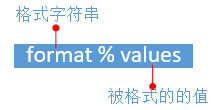格式字元串：使用%s(對應值為字元串)，%d(對應值為數字)等等，還可以在中間插入修飾符%03d。

被格式的值：只能是一個對象，可以是元組或是字典。

``````name = "Tom"
age = 18
print("%s is %d age." % (name,age))
# 輸出結果如下：
Tom is 18 age.
``````

format格式化：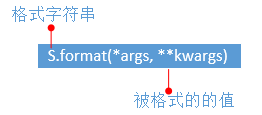格式字元串：使用花括弧{ }, 花括弧裡面可以使用修飾符。

被格式的值：*args為可變位置參數，**kwargs為可變關鍵字參數。

``````# 位置傳參
print("IP={} PORT={}".format('8.8.8.8',53))  # 位置傳參
print("{Server}: IP={1} PORT={0}".format(53, '8.8.8.8', Server='DNS Server'))  # 位置和關鍵字傳參傳參

# 輸出結果如下：
IP=8.8.8.8 PORT=53
DNS Server: IP=8.8.8.8 PORT=53
``````
``````# 浮點數
print("{}".format(0.123456789))
print("{:f}".format(0.123456789))    #  小數點預設為6位
print("{:.2f}".format(0.123456789))  # 取小數點後兩位
print("{:15}".format(0.123456789))   # 寬度為15，右對齊

# 輸出結果如下：
0.123456789
0.123457     # 為什麼是這個值？大於5要進位
0.12
0.123456789  # 左邊有4個空格
``````
• 其他常用函數

``````str = "DianDiJiShu"
print(str.upper())  # 字母全部轉化為大寫
print(str.lower())  # 字母全部轉化為小寫

# 輸出結果如下：
DIANDIJISHU
diandijishu
``````

### 2.2.4 bytes 位元組

`bytes ``bytearray`從python3引入的兩種數據類型。

• 把人類的語言編碼成機器能夠識別的語言，通常叫做編碼（字元串轉換為ASCII碼）。
• 把機器的語言解碼成人類能夠識別的語言，通常叫做解碼（ASCII碼轉換為字元串）。

ASCII碼表常用的必須牢記(整理部分)：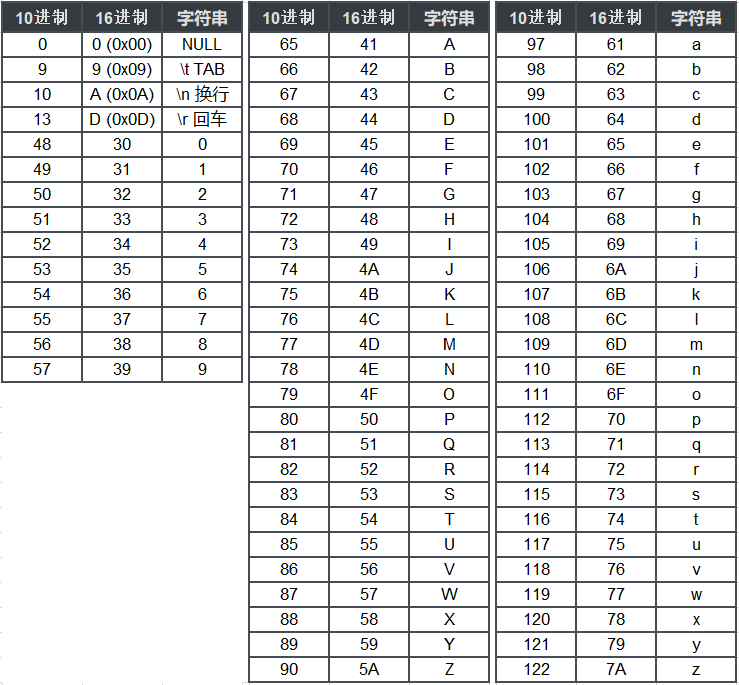``````鏈接：https://pan.baidu.com/s/1fWVl57Kqmv-tkjrDKwPvSw 提取碼：tuyz
``````

``````# 二進位
0000 0010  # 一個位元組bytes

# 16進位，機器基本都是顯示16進位
0x2
``````

bytes 是不可變類型

``````bytes()     # 空bytes，一旦創建不可改變
bytes(int)  # 指定位元組的大小，用0填充
bytes(iterable_of_ints)  # [0.255]整數的可迭代對象
bytes(string, encoding[, errors])  # 等價於string.encoding(),字元串編碼成位元組
bytes(bytes_or_buffer)  # 複製一份新的位元組對象
``````
• 初始化

``````b1 = bytes()
b2 = bytes(range(97,100))
b3 = bytes(b2)
b4 = bytes('123',encoding='utf-8')
b5 = b'ABC'
b6 = b'\xe4\xbd\xa0\xe5\xa5\xbd'.decode('utf-8')
print(b1, b2, b3, b4, b5, b6, sep='\n')

# 輸出結果如下：
b''
b'abc'
b'abc'
b'123'
b'ABC'
你好
``````

### 2.2.5 bytearray 位元組數組

bytearray 是可變數組，可以進行增刪改操作，類似列表。

``````bytearray()  # 空bytearray,可改變
bytearray(iterable_of_ints)  # [0.255]整數的可迭代對象
bytearray(string, encoding[, errors])  # 等價於string.encoding(),字元串編碼成位元組
bytearray(bytes_or_buffer)   # 複製一份新的位元組數組對象
bytearray(int)  # 指定位元組的大小，用0填充
``````
• 增刪改

``````# 初始化
b = bytearray()
print(b)
# 輸出結果如下：
bytearray(b'')
#--------------------------
# 增加元素對象
b.append(97)
print(b)
b.extend([98,99])
print(b)
# 輸出結果如下：
bytearray(b'a')
bytearray(b'abc')
#--------------------------
# 插入元素對象
b.insert(0,65)
print(b)
# 輸出結果如下：
bytearray(b'Aabc')
#--------------------------
# 刪除元素對象
b.pop()
print(b)
# 輸出結果如下：
bytearray(b'Aab')
``````• 前端作為互聯網時代直接觸達用戶的視窗，大到我們每天瀏覽到的網站，小到一次點擊按鈕的頁面，前端無處不在。並且在產品的眾多開發環節之中，最能讓用戶直觀感受到的就是前端開發。因而前端行業的廣闊發展前景也吸引了不少人的轉業學習。那麼，零基礎如何學好前端呢？有什麼建議嗎？本文將為初學者詳細解答一下這兩個問題， ...
• 正常我們寫一個左右兩列，左側一列放置圖片的html，如下所示： <!DOCTYPE html> <html lang="en"> <head> <meta charset="UTF-8"> <title>Title</title> <style> .container{ background-col ...
• 在vue項目中，為了減少首屏載入的時間，通常會開啟路由的懶載入。路由懶載入配合gizp確實能幫助我們大大的加快首屏的載入時間。 然而，路由懶載入會使得我們在第一次打開一個新頁面的時候，會有一個載入時間。如果在這個時候我們沒有一個提示的話，給人的感覺會是好像我點了頁面跳轉但是沒反應。所以，這個時候我們 ...
• 最詳細的css3選擇器解析 選擇器是什麼？ 比較官方的解釋：在 CSS 中，選擇器是一種模式，用於選擇需要添加樣式的元素。 最常見的 CSS 選擇器是元素選擇器。換句話說，文檔的元素就是最基本的選擇器。 看代碼，元素選擇器就是這個： h1作為一個元素標簽，是最基本的選擇器，這樣可以對h1標簽設置屬性 ...
• 首先保證路徑正確，引入ttf字體，沒有生效 使用線上工具把ttf轉為更多常用的字體格式 網址：https://www.fontke.com/tool/convfont/ css里補全格式： 生效了 ...
• 導讀 轉載自 "冪等性如何實現？深入瞭解一波！！！" 現在這個時代大家可能最關心的就是錢了，那麼有沒有想過你銀行轉賬給你沒有一次是轉多的，要麼失敗，要麼成功，為什麼不能失誤一下多轉一筆呢？醒醒吧年輕人，別做夢了，做銀行的能那麼傻x嗎？ 今天我們就來談一談為什麼銀行轉賬不能多給我轉一筆？關乎到錢的問題 ...
• 功能介紹：黑貓關鍵詞URL採集工具 Pro v1.0 批量關鍵詞自動搜索採集 自動去除垃圾二級泛解析功能變數名稱 可設置是否保存功能變數名稱或者url 聯繫客服QQ:944520563 ...

• 一：背景 1. 講故事 如果你常翻看FCL的源碼，你會發現這裡面有不少方法藉助了C/C++的力量讓C#更快更強悍,如下所示： [DllImport("QCall", CharSet = CharSet.Unicode)] [SecurityCritical] [SuppressUnmanagedCo ...
• 上一篇(https://www.cnblogs.com/meowv/p/12966092.html)文章使用AutoMapper來處理對象與對象之間的映射關係，本篇主要圍繞定時任務和數據抓取相關的知識點並結合實際應用，在定時任務中迴圈處理爬蟲任務抓取數據。 開始之前可以刪掉之前測試用的幾個Hello ...
• 首先創建實體類 1 public class MacState 2 { 3 /// <summary> 4 /// 請求狀態 5 /// </summary> 6 public string success { get; set; } 7 /// <summary> 8 /// 錯誤信息 9 /// ...
• 0. 前言 前幾天FreeSql的作者向我推薦了FreeSql框架，想讓我幫忙寫個文章介紹一下。嗯，想不到我也能帶個貨了。哈哈，開個玩笑～看了下覺得設計的挺有意思的，所以就謝了這篇文章。 簡單介紹一下，FreeSql 是NCC組織的沙盒級項目，是一款功能強大的 ORM 組件，支持 .NET Core ...
• 0. 前言 這是一個新的系列，名字是《ASP.NET Core 入門到實戰》。這個系列主講ASP.NET Core MVC，輔助一些前端的基礎知識（能用來實現我們需要的即可，並非主講）。同時這個系列也會在後續介紹ASP.NET Core 平臺的其它類型的項目，並帶領大家以各個類型的項目為主要架構開發 ...
• 我寫了一個Winform測試程式，用的System.Timers.Timer，在事件里，設置label1.Text，然後，居然句柄泄漏、用戶對象泄漏！ 百思不得其解，最後換成System.Windows.Forms.Timer，居然不泄漏了！ 最近睡眠不足，哪怕一個很小的問題，隨便搞搞，都半夜了！ ...
• leetcode-7. 整數反轉。 給出一個 32 位的有符號整數，你需要將這個整數中每位上的數字進行反轉。 示例 1: 輸入: 123 輸出: 321 示例 2: 輸入: -123 輸出: -321 示例 3: 輸入: 120 輸出: 21 註意: 假設環境只能存儲得下 32 位的有符號整數，則其 ...
• 1. Java 虛擬機是什麼？ 1.1 虛擬機 虛擬機：虛擬的電腦，一個用來執行虛擬電腦指令的軟體。 虛擬機分為系統虛擬機和程式虛擬機。 系統虛擬機：提供一個可運行完整操作系統的軟體平臺，如 Visual Box、VMware。 程式虛擬機：專門執行單個程式的，典型代表 Java 虛擬機。Jav ...
• 前言 - strlen 概述 無意間掃到 glibc strlen.c 中代碼, 久久不能忘懷. 在一無所知的編程生涯中又記起點點滴滴: 編程可不是兒戲 ❀, 有些難, 也有些不捨. 隨軌跡一同重溫, 曾經最熟悉的 strlen 手感吧 ~ /* Copyright (C) 1991-2020 Fr ...
• 背景 隊列[Queue]：是一種限定僅在表頭進行刪除操作，僅在表尾進行插入操作的線性表；即先進先出（FIFO-first in first out）:最先插入的元素最先出來。 本文通過編碼實現鏈式隊列類，並模擬一個有趣的應用，能夠幫助我們對鏈式隊列有更深度的理解。 基本概念 結點 每個元素，除了存儲 ...